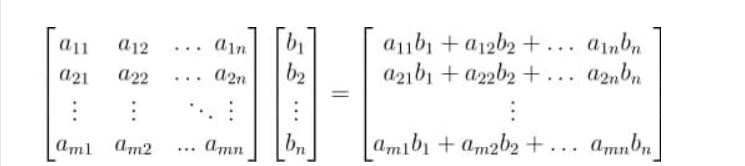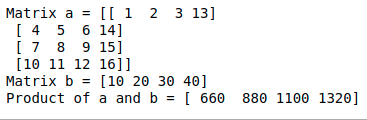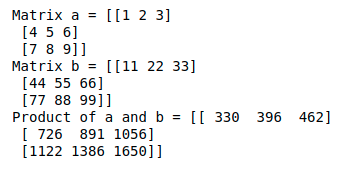GeeksforGeeks App
Open AppBrowser
Continue

# Parallel matrix-vector multiplication in NumPy

In this article, we will discuss how to do matrix-vector multiplication in NumPy.

## Matrix multiplication with Vector

For a matrix-vector multiplication, there are certain important points:

• The end product of a matrix-vector multiplication is a vector.
• Each element of this vector is obtained by performing a dot product between each row of the matrix and the vector being multiplied.
• The number of columns in the matrix is equal to the number of elements in the vector.```# a and b are matrices
prod = numpy.matmul(a,b)```

For matrix-vector multiplication, we will use np.matmul() function of NumPy, we will define a 4 x 4 matrix and a vector of length 4.

## Python3

 `import` `numpy as np`` ` `a ``=` `np.array([[``1``, ``2``, ``3``, ``13``],``              ``[``4``, ``5``, ``6``, ``14``],``              ``[``7``, ``8``, ``9``, ``15``],``              ``[``10``, ``11``, ``12``, ``16``]])`` ` `b ``=` `np.array([``10``, ``20``, ``30``, ``40``])`` ` `print``(``"Matrix a ="``, a)``print``(``"Matrix b ="``, b)``print``(``"Product of a and b ="``,``      ``np.matmul(a, b))`

Output:## Matrix multiplication with another Matrix

We use the dot product to do matrix-matrix multiplication. We will use the same function for this also.

`prod = numpy.matmul(a,b)  # a and b are matrices`

For a matrix-matrix multiplication, there are certain important points:

• The number of columns in the first matrix should be equal to the number of rows in the second matrix.
• If we are multiplying a matrix of dimensions m x n with another matrix of dimensions n x p, then the resultant product will be a matrix of dimensions m x p

We will define two 3 x 3 matrix:

## Python3

 `import` `numpy as np`` ` `a ``=` `np.array([[``1``, ``2``, ``3``],``              ``[``4``, ``5``, ``6``],``              ``[``7``, ``8``, ``9``]])`` ` `b ``=` `np.array([[``11``, ``22``, ``33``],``              ``[``44``, ``55``, ``66``],``              ``[``77``, ``88``, ``99``]])`` ` `print``(``"Matrix a ="``, a)``print``(``"Matrix b ="``, b)``print``(``"Product of a and b ="``, np.matmul(a, b))`

Output:My Personal Notes arrow_drop_up